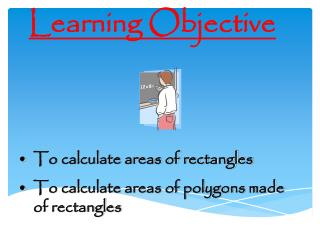# Learning Objective - PowerPoint PPT PresentationDownload PresentationLearning Objective

Learning ObjectiveDownload Presentation## Learning Objective

- - - - - - - - - - - - - - - - - - - - - - - - - - - E N D - - - - - - - - - - - - - - - - - - - - - - - - - - -
##### Presentation Transcript

1. Learning Objective • To calculate areas of rectangles • To calculate areas of polygons made of rectangles

2. What is area measured in? Area is measured in SQUARE CENTIMETRES. A square centimetre is a square in which all the sides measure 1 cm. Area is also measured in SQUARE METRES. A square metre is a square in which all the sides measure 1 metre.

3. 1 cm 1 cm Area is the measure of how much space a shape takes up. We measure it in squares such as square centimetres or metres etc. This rectangle takes up 28 squares. It has an area of 28 square centimetres 28 cm2 1 2 3 4 5 6 7 8 9 10 11 12 13 14 15 16 17 18 19 20 21 22 23 24 25 26 27 28

4. It could take a long time to cover shapes in squares. Luckily there is an quicker way. 7 cm 4 cm × = 28 cm2

5. Use this formulae to find the area of rectangles. length breadth Area of a rectangle = length × breadth

6. 5 cm 2 cm 3 cm 7 cm 7 m 7 m Can you find the areas of these rectangles? 15 cm2 14 cm2 49 m2

7. Can you think of a way to find the area of this shape? 5 cm 6 cm 7 cm 3 cm 12 cm

8. Split the shape into rectangles? 5 cm 6 cm 7 cm 3 cm 12 cm

9. Find the area of each rectangle? 5 cm 5 × 6 = 30 cm2 7 × 3 = 21 cm2 6 cm 7 cm 3 cm

10. Add the areas together to find the area of the complete shape? 30 + 21 = 51 cm2 30 cm2 21 cm2

11. 2 cm 4 cm 11 cm 5 cm Can you find the areas of these shapes? 6 m 22 m2 3 m 4 m 43 cm2 4 m

12. Here is a challenge can you work out the area of this shape with a hole in it? 2 cm 5 cm 5 cm 10 cm Clue: Take the area of the hole from the area of the whole! 50 cm2 – 10 cm2 = 40 cm2

13. Remember: Area of a rectangle = length × breadth length breadth Split more complicated shapes into rectangles and find the area of each rectangle then add them together. Over to you.

14. OMA Find Fractions of a number

15. Learning Objective • Calculate the area of a right angled triangle by considering it half a rectangle

16. Area of triangles

17. What’s the area of this rectangle? 10 cm 6 cm 60 cm2

18. What’s the area of the red triangle? 10 cm 6 cm 30 cm2

19. Area of a triangle height length Area = ½ length x height

20. What’s the area? 4 cm 8 cm 16 cm2 ½ of 8 x 4 =

21. What’s the area? 8 cm 32 cm2 8 cm

22. What’s the area? 8 cm 12 cm2 3 cm

23. What’s the area? 2 cm 14 cm 14 cm2

24. What’s the area? 15cm 20cm 150 cm2

25. What’s the area? 20 cm 8 cm 80 cm2

26. What if the triangle doesn’t have a right angle?

27. Split it up! 4 cm 20 cm

28. Split it up! 4 cm 10 cm 10 cm 20 cm (½ of 10 x 4) + ( ½ of 10 x 4) = 20 + 20 = 40 ½ of 20 x 4 = 40

29. What’s the area? 10 cm 40 cm2 8 cm

30. OMA Find Fractions of a number

31. Brain Train LO: To recognise and draw reflections of shapes.

32. Shape 1 • Look at this shape. • Can you spot the vertical reflection of the shape on the next slide? Hold up the correct letter when asked.

33. A B C D

34. Congratulations!The correct answer is B!

35. Shape 2 • Look at this shape. • Can you spot the vertical reflection of the shape on the next slide? Hold up the correct letter when asked.

36. A B C D

37. Congratulations!The correct answer is A!

38. Shape 3 • Look at this shape. • Can you spot the vertical reflection of the shape on the next slide? Hold up the correct letter when asked.

39. A B C D

40. Congratulations!The correct answer is C!

41. Shape 4 • Look at this shape. • Can you spot the horizontal reflection of the shape on the next slide? Hold up the correct letter when asked.

42. A B C D

43. Congratulations!The correct answer is D!

44. Shape 5 • Look at this shape. • Can you spot the horizontal reflection of the shape on the next slide? Hold up the correct letter when asked.

45. A B C D

46. Congratulations!The correct answer is B!

47. Shape 6 • Look at this shape. • Can you spot the horizontal reflection of the shape on the next slide? Hold up the correct letter when asked.

48. A B C D

49. Congratulations!The correct answer is A!

50. Shape 7 • Look at this shape. • Can you spot the diagonal reflection of the shape on the next slide? Hold up the correct letter when asked.